Home Practice
For learners and parents For teachers and schools
Textbooks
Full catalogue
Pricing SupportLog in

We think you are located in United States. Is this correct?

# 13.7 Applications of electrochemistry

## 13.7 Applications of electrochemistry (ESCRP)

Electrochemistry has a number of different uses, particularly in industry. The principles of cells are used to make electrical batteries. In science and technology, a battery is a device that stores chemical energy and makes it available in an electrical form. Batteries are made of electrochemical devices such as one or more galvanic cells or fuel cells. Batteries have many uses including in:

A fuel cell converts the chemical potential energy produced by the oxidation of fuels (e.g. hydrogen gas, hydrocarbons, alcohols) into electrical energy.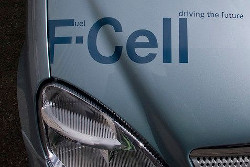• torches

• electrical appliances such as cellphones (long-life alkaline batteries)

• digital cameras (lithium batteries)

• hearing aids (silver-oxide batteries)

• digital watches (mercury/silver-oxide batteries)

• military applications (thermal batteries)

In this section we are going to look at a few examples of the uses of electrochemistry in industry.

### Electroplating (ESCRQ)

The electrolytic cell can be used for electroplating.

Electroplating

The process of coating an electrically conductive object with a thin layer of metal using an electrical current.

Electroplating occurs when an electrically conductive object is coated with a layer of metal using electrical current. Sometimes, electroplating is used to give a metal particular properties or for aesthetic reasons:

• corrosion protection

• abrasion and wear resistance

• the production of jewellerya) An electroplated piece of aluminium artwork and b) a wax stool electroplated in copper.

Electro-refining (also sometimes called electrowinning) is electroplating on a large scale. Copper plays a major role in the electrical industry as it is very conductive and is used in electric cables. One of the problems though is that copper must be pure if it is to be an effective current carrier. One of the methods used to purify copper, is electrowinning (copper ore is processed into impure blister copper, which is then deposited as pure copper through electroplating). The copper electrowinning process is as follows:

1. A bar of $$\color{copperone}{\textbf{impure}}$$ copper containing other metallic impurities acts as the $$\color{blue}{\textbf{anode}}$$.

2. The $$\color{red}{\textbf{cathode}}$$ is made up of $$\color{coppertwo}{\textbf{pure}}$$ copper with few impurities.

3. The electrolyte is a solution of aqueous $$\text{CuSO}_{4}$$ and $$\text{H}_{2}\text{SO}_{4}$$.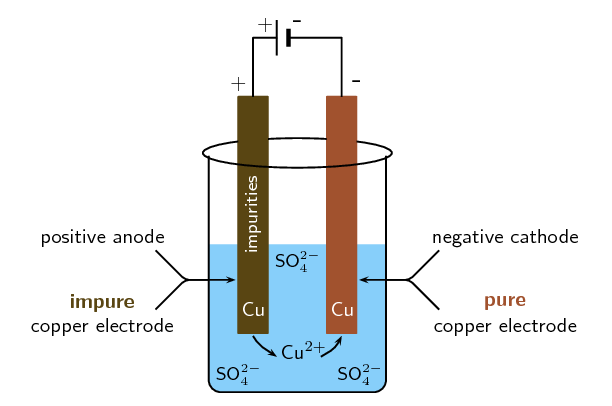Figure 13.12: A simplified diagram to illustrate what happens during the electrowinning of copper.
4. When current passes through the cell, electrolysis takes place:

• The $$\color{copperone}{\textbf{impure copper anode}}$$ oxidises to form $$\text{Cu}^{2+}$$ ions in solution. The $$\color{blue}{\textbf{anode decreases in mass}}$$.

$$\color{copperone}{\textbf{Cu(s)}} \to$$ $$\text{Cu}^{2+}(\text{aq}) + 2\text{e}^{-}$$

• At the cathode reduction of positive copper ions takes place to produce $$\color{coppertwo}{\textbf{pure copper metal}}$$. The $$\color{red}{\textbf{cathode}}$$ $$\color{red}{\textbf{increases in mass}}$$.

$$\text{Cu}^{2+}(\text{aq}) + 2\text{e}^{-}$$ $$\to \color{coppertwo}{\textbf{Cu(s)}}$$ ($$>$$99% purity)

5. The other metal impurities do not dissolve ($$\text{Au}(\text{s})$$, $$\text{Ag}(\text{s})$$) and form a solid sludge at the bottom of the tank or remain in solution ($$\text{Zn}(\text{aq})$$, $$\text{Fe}(\text{aq})$$ and $$\text{Pb}(\text{aq})$$) in the electrolyte.

temp text

### The chloralkali industry (ESCRR)

The chlorine-alkali (chloralkali) industry is an important part of the chemical industry, which produces chlorine and sodium hydroxide through the electrolysis of the raw material brine. Brine is a saturated solution of sodium chloride ($$\text{NaCl}$$) that is obtained from natural salt deposits.

Brine

A saturated aqueous solution of sodium chloride.

Remember that electrolytic cells are used to transform reactants into products by turning electric current into chemical potential energy.

The products of the chloralkali industry have a number of important uses:

Chlorine is used:

• to purify water

• as a disinfectant

• in the production of:

• hypochlorous acid (used to kill bacteria in drinking water)

• paper, food

• antiseptics, insecticides, medicines, textiles, laboratory chemicals

• paints, petroleum products, solvents, plastics (such as polyvinyl chloride)

Sodium hydroxide (also known as 'caustic soda') is used to:

• make soap and other cleaning agents

• purify bauxite (the ore of aluminium)

• make paper

• make rayon (artificial silk)

One of the problems of producing chlorine and sodium hydroxide is that when they are produced together the chlorine combines with the sodium hydroxide to form chlorate ($$\text{ClO}^{-}$$) and chloride ($$\text{Cl}^{-}$$) ions. This leads to the production of sodium chlorate, $$\text{NaClO}$$, a component of household bleach.

To overcome this problem the chlorine and sodium hydroxide must be separated from each other so that they don't react. There are three industrial processes that have been designed to overcome this problem. All three methods involve electrolytic cells.

1. The Mercury Cell

In the mercury-cell (Figure 13.13):

• The anode is a carbon electrode suspended from the top of a chamber.

• The cathode is liquid mercury that flows along the floor of this chamber.

• The electrolyte is brine ($$\text{NaCl}$$ solution) that is passed through the chamber.

• When an electric current is applied to the circuit, chloride ions in the electrolyte are $$\color{blue}{\text{oxidised at the anode}}$$ to form chlorine gas.

$$\color{blue}{\text{2Cl}^{-}\text{(aq)} \to \text{Cl}_{2} \text{(g) + 2e}^{-}}$$

• At the same time sodium ions are $$\color{red}{\text{reduced at the anode}}$$ to solid sodium. The solid sodium dissolves in the mercury making a sodium/mercury amalgam.

$$\color{red}{\text{Na}^{+}\text{(aq) + Hg(ℓ) + e}^{-} \to \text{Na(Hg)}}$$

• The amalgam is poured into a separate vessel, where it decomposes into sodium and mercury.

• The sodium reacts with water in the vessel and produces sodium hydroxide and hydrogen gas, while the mercury returns to the electrolytic cell to be used again.

$$\color{red}{\text{2Na(Hg) + 2H}_{2}\text{O(ℓ)} \to \text{2NaOH(aq) + H}_{2}\text{(g) + Hg(ℓ)}}$$

In a mercury cell the sodium dissolves in the liquid mercury to form a liquid amalgam of the two metals. This separates the $$\text{Cl}^{-}$$ and $$\text{Na}^{+}$$ ions.

This method only produces a fraction of the chlorine and sodium hydroxide that is used by industry as it has certain disadvantages:

• mercury is expensive and toxic

• some mercury always escapes with the brine that has been used

• mercury reacts with the brine to form mercury(II) chloride

• the mercury cell requires a lot of electricity

• although the chlorine gas produced is very pure, mercury has to be removed from the sodium hydroxide and hydrogen gas mixture.

In the past the effluent was released into lakes and rivers, causing mercury to accumulate in fish and other animals feeding on the fish. Today, the brine is treated before it is discharged so that the environmental impact is lower.

2. The Diaphragm Cell

In the diaphragm-cell (Figure 13.14):

• a porous diaphragm divides the electrolytic cell into an anode compartment and a cathode compartment

• brine is introduced into the anode compartment and flows through the diaphragm into the cathode compartment

• an electric current is passed through the brine causing the salt's chlorine ions and sodium ions to move to the electrodes

• Chlorine gas is produced at the $$\color{blue}{\text{anode}}$$

$$\color{blue}{\text{2Cl}^{-}\text{(aq) + 2e}^{-} \to \text{Cl}_{2}\text{(g)}}$$

• At the $$\color{red}{\text{cathode}}$$, sodium ions react with water forming caustic soda ($$\text{NaOH}$$) and hydrogen gas.

$$\color{red}{\text{2Na}^{+}\text{(aq) + 2H}_{2}\text{O(ℓ) + e}^{-} \to \text{2NaOH(aq) + H}_{2}\text{(g)}}$$

• Some $$\text{NaCl}$$ salt remains in the solution with the caustic soda and can be removed at a later stage.

The advantages of the diaphragm cell are:

• uses less energy than the mercury cell

• does not contain toxic mercury

• the sodium hydroxide is much less concentrated and not as pure

• the chlorine gas often contains oxygen gas as well

• the process is less cost-effective as the sodium hydroxide solution needs to be concentrated and purified before it can be used

To separate the chlorine from the sodium hydroxide, the two half-cells were traditionally separated by a porous asbestos diaphragm, which needed to be replaced every two months.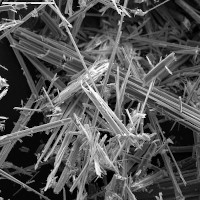This was damaging to the environment, as large quantities of asbestos had to be disposed. Asbestos is toxic to humans, and causes cancer and lung problems. Today, the asbestos is being replaced by other polymers, which do not need to be replaced as often, and are not toxic.

3. The Membrane Cell

The membrane cell (Figure 13.15) is very similar to the diaphragm cell, and the same reactions occur. The main differences are:

• the two electrodes are separated by an ion-selective membrane, rather than by a diaphragm

• the membrane structure allows cations to pass through it between compartments of the cell but does not allow anions to pass through (this has nothing to do with the size of the pores, but rather with the charge on the ions)

• brine is pumped into the anode compartment, and only the $$\color{red}{\text{positively charged}}$$ $$\color{red}{\text{sodium ions}}$$ pass into the cathode compartment, which contains pure water

• At the $$\color{blue}{\textbf{positively charged anode}}$$, $$\text{Cl}^{-}$$ ions from the brine are oxidised to $$\text{Cl}_{2}$$ gas.

$$\color{blue}{\text{2Cl}^{-}\text{(aq)} \to \text{Cl}_{2}\text{(g) + 2e}^{-}}$$

• At the $$\color{red}{\textbf{negatively charged cathode}}$$, hydrogen ions in the water are reduced to hydrogen gas.

$$\color{red}{\text{2H}_{2}\text{O(ℓ) + 2e}^{-} \to \text{H}_{2}\text{(g) + 2OH}^{-}}$$

• The $$\text{Na}^{+}$$ ions flow through the membrane to the cathode compartment and react with the remaining hydroxide ($$\text{OH}^{-}$$) ions from the water to form sodium hydroxide ($$\text{NaOH}$$).

$$\color{red}{\text{Na}^{+}\text{(aq) + OH}^{-}\text{(aq)} \to \text{NaOH(aq)}}$$

• The chloride ions cannot pass through the membrane, so the chlorine does not come into contact with the sodium hydroxide in the cathode compartment. The sodium hydroxide is removed from the cell. The overall equation is as follows:

$$2\text{NaCl}(\text{aq}) + 2\text{H}_{2}\text{O}(\text{ℓ})$$ $$\to$$ $$\text{Cl}_{2}(\text{g}) + \text{H}_{2}(\text{g}) + 2\text{NaOH}(\text{g})$$

The advantages of using this method are:

• the sodium hydroxide that is produced is very pure because it is kept separate from the sodium chloride solution

• the sodium hydroxide has a relatively high concentration

• this process uses the least electricity of all three cells

• the cell is cheaper to operate than the other two cells

• the cell does not contain toxic mercury or asbestos

temp text

## The chloralkali industry

Textbook Exercise 13.10

Refer to the flow diagram, which shows the reactions that take place in the membrane cell, and then answer the questions that follow.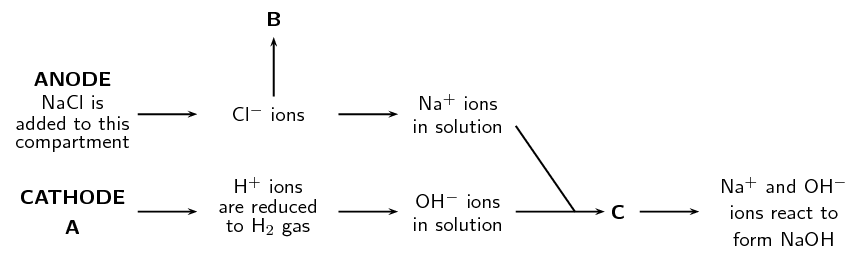What liquid is present in the cathode compartment at A?

Water and dilute $$\text{NaOH}$$

Identify the gas that is produced at B.

Chlorine gas

Explain one feature of this cell that allows the $$\text{Na}^{+}$$ and $$\text{OH}^{-}$$ ions to react at C.

This type of cell has an ion permeable membrane that allows the positive $$\text{Na}^{+}$$ ions to move from the anode compartment to the cathode compartment and interact with the $$\text{OH}^{-}$$ ions.

Give a balanced equation for the reaction that takes place at C.

$$\text{Na}^{+}(\text{aq}) + \text{OH}^{-}(\text{aq})$$ $$\to$$ $$\text{NaOH}(\text{aq})$$

Summarise what you have learnt about the three types of cells in the chloralkali industry by completing the table below:

 Mercury cell Diaphragm cell Membrane cell Main raw material Mechanism of separating $$\text{Cl}_{2}$$ and $$\text{NaOH}$$ Anode reaction Cathode reaction Purity of NaOH produced Purity of $$\text{Cl}_{2}$$ produced Energy consumption Environmental impact Cost of production
 Mercury cell Diaphragm cell Membrane cell Main raw material brine brine brine Mechanism of separating $$\text{Cl}_{2}$$ and $$\text{NaOH}$$ reduction of $$\text{Na}^{+}$$ to $$\text{Na}$$ in $$\text{Hg}$$ amalgam use of a porous diaphragm use of a membrane (charge specific) Anode reaction $$\text{Cl}^{-}$$ ions $$\to$$ $$\text{Cl}_{2}$$ gas $$\text{Cl}^{-}$$ ions $$\to$$ $$\text{Cl}_{2}$$ gas $$\text{Cl}^{-}$$ ions $$\to$$ $$\text{Cl}_{2}$$ gas Cathode reaction $$\text{Na}^{+}$$ ions $$\to$$ $$2\text{Na}(\text{Hg})$$ $$2\text{Na}(\text{Hg}) + 2\text{H}_{2}\text{O}(\text{ℓ})$$ $$\to$$ $$2\text{NaOH}(\text{aq}) + \text{H}_{2}(\text{g})$$ $$2\text{Na}^{+}(\text{aq}) + \text{H}_{2}\text{O}(\text{ℓ})$$ $$\to$$ $$2\text{NaOH}(\text{ℓ}) + \text{H}_{2}(\text{g})$$ $$2\text{Na}^{+}(\text{aq}) + \text{H}_{2}\text{O}(\text{ℓ})$$ $$\to$$ $$2\text{NaOH}(\text{ℓ}) + \text{H}_{2}(\text{g})$$ Purity of NaOH low purity medium purity low concentration high purity Purity of $$\text{Cl}_{2}$$ high purity mixed with $$\text{O}_{2}$$ mixed with $$\text{O}_{2}$$ Energy consumption high less than the mercury cell low energy Environmental impact high lower than the mercury cell lower than the diaphragm and mercury cell Cost of production expensive expensive, as product must be purified low production cost

The diagram below shows the sequence of steps that take place in the mercury cell.Name the 'raw material' in step 1.

Aqueous sodium chloride ($$\text{NaCl}$$, brine)

Give the chemical equation for the reaction that produces chlorine gas in step 2.

$$2\text{Cl}^{-}(\text{aq})$$ $$\to$$ $$\text{Cl}_{2}(\text{g}) + 2\text{e}^{-}$$

What other product is formed in step 2.

sodium mercury amalgam

Name the reactants in step 4.

sodium mercury amalgam and water

Approximately $$\text{30}$$ million tonnes of chlorine are used throughout the world annually. Chlorine is produced industrially by the electrolysis of brine. The diagram represents a membrane cell used in the production of $$\text{Cl}_{2}$$ gas.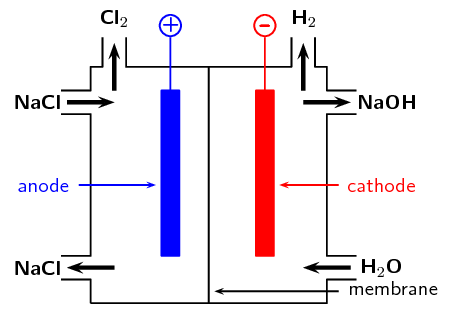What ions are present in the electrolyte in the left hand compartment of the cell?

Chloride ions ($$\text{Cl}^{-}$$) and sodium ions ($$\text{Na}^{+}$$).

Give the equation for the reaction that takes place at the anode.

$$2\text{Cl}^{-}(\text{aq})$$ $$\to$$ $$\text{Cl}_{2}(\text{g}) + 2\text{e}^{-}$$

Give the equation for the reaction that takes place at the cathode and forms a gas.

$$2\text{H}_{2}\text{O}(\text{ℓ}) + 2\text{e}^{-}$$ $$\to$$ $$\text{H}_{2}(\text{g}) + 2\text{OH}^{-}(\text{aq})$$

What ion passes through the membrane while these reactions are taking place?

Sodium ions

Chlorine is used to purify drinking water and swimming pool water. The substance responsible for this process is the weak acid, hypochlorous acid ($$\text{HOCl}$$). One way of putting $$\text{HOCl}$$ into a pool is to bubble chlorine gas through the water. Give an equation showing how bubbling $$\text{Cl}_{2}(\text{g})$$ through water produces $$\text{HOCl}$$.

$$\text{Cl}_{2}(\text{g}) + \text{H}_{2}\text{O}(\text{ℓ})$$ $$\to$$ $$\text{HOCl}(\text{aq}) + \text{HCl}(\text{aq})$$

A common way of treating pool water is by adding 'granular chlorine'. Granular chlorine consists of the salt calcium hypochlorite, $$\text{Ca}(\text{OCl})_{2}$$. Give an equation showing how this salt dissolves in water. Indicate the phase of each substance in the equation.

(IEB Paper 2, 2003)

$$\text{Ca}(\text{OCl})_{2}(\text{s})$$ $$\to$$ $$\text{Ca}^{2+}(\text{aq}) + 2\text{OCl}^{-}(\text{aq})$$

### The extraction of aluminium (ESCRS)

Aluminium is a commonly used metal in industry, where its properties of being both light and strong can be utilised. It is used in the manufacture of products such as aeroplanes and motor cars. The metal is present in deposits of bauxite. Bauxite is a mixture of silicas, iron oxides and hydrated alumina ($$\text{Al}_{2}\text{O}_{3}$$.x$$\text{H}_{2}\text{O}$$).

Bauxite is a rock that contains a large amount of aluminum oxide ($$\text{Al}_{2}\text{O}_{3}$$) and aluminium hydroxide ($$\text{Al}(\text{OH})_{3}$$) as well as many other aluminium containing minerals. Bauxite is the richest source of aluminum when compared with any other common rock, and is the best aluminum ore.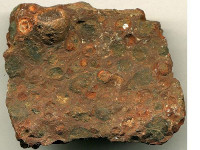Electrolysis can be used to extract aluminium from bauxite. The process described below produces 99% pure aluminium:

1. Aluminium is melted along with cryolite ($$\text{Na}_{3}\text{AlF}_{6}$$) which acts as the electrolyte. Cryolite helps to lower the melting point and dissolve the ore.

2. The $$\color{blue}{\text{carbon rod anode}}$$ provides a site for the oxidation of $$\text{O}^{2-}$$ and $$\text{F}^{-}$$ ions. Oxygen and fluorine gas are given off at the anode and also result in anode consumption.

$$\color{blue}{\text{2O}^{2-}\text{(aq)} \to \text{O}_{2}\text{(g) + 4e}^{-}}$$

$$\color{blue}{\text{2F}^{-}\text{(aq)} \to \text{F}_{2}\text{(g) + 2e}^{-}}$$

3. At the $$\color{red}{\text{cathode cell lining}}$$, the $$\text{Al}^{3+}$$ ions are reduced and metal aluminium deposits on the lining.

$$\color{red}{\text{Al}^{3+}\text{(aq) + 3e}^{-} \to \text{Al(s)}}$$ (99% purity)

4. The $$\text{AlF}_{6}^{3-}$$ electrolyte is stable and remains in its molten state.

The overall reaction is as follows:

$$2\text{Al}_{2}\text{O}_{3}(\text{s})$$ $$\to$$ $$4\text{Al}(\text{s}) + 3\text{O}_{2}(\text{g})$$

The only problem with this process is that the reaction is endothermic and large amounts of electricity are needed to drive the reaction. The process is therefore very expensive.

temp text